# Geometric optics

11 de Jan de 2012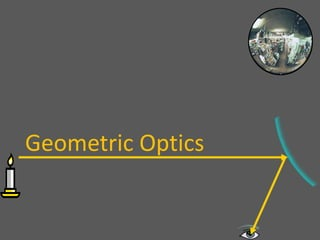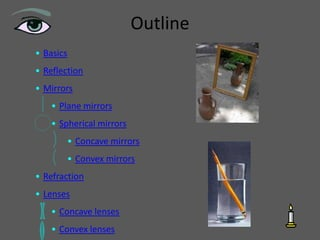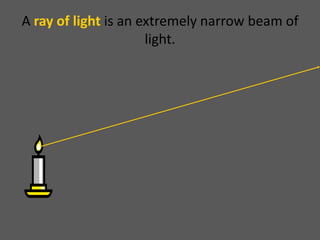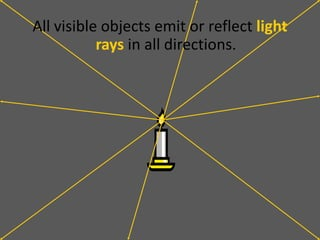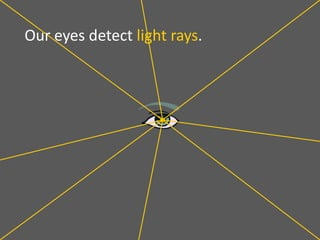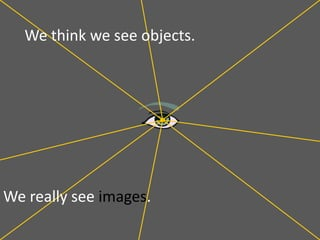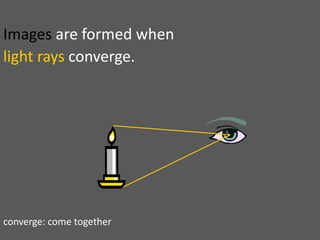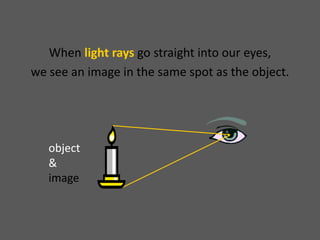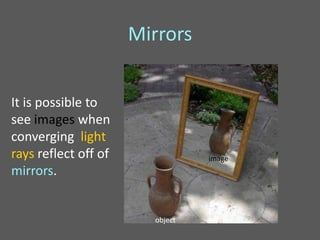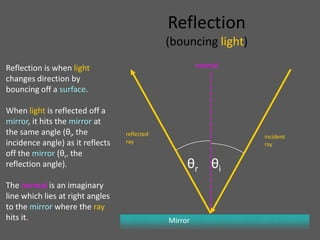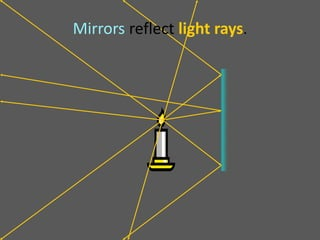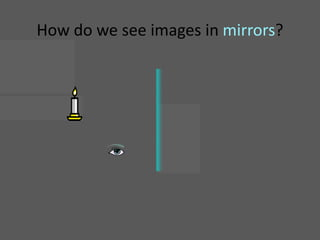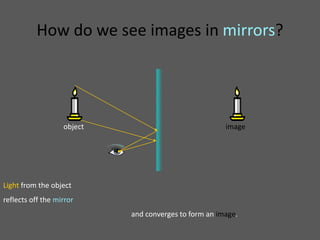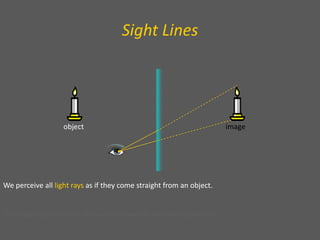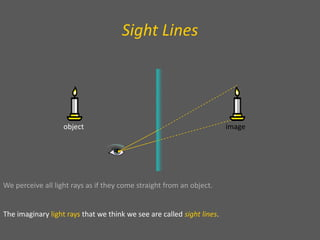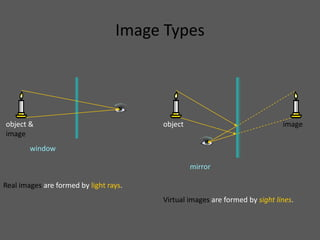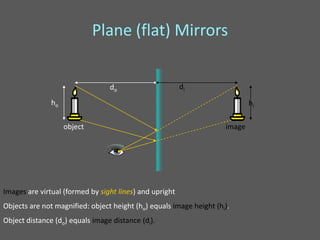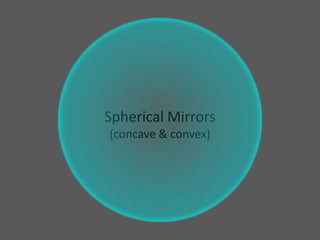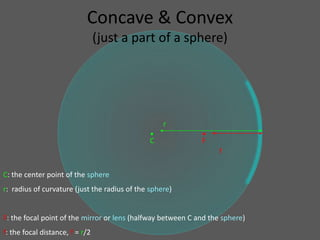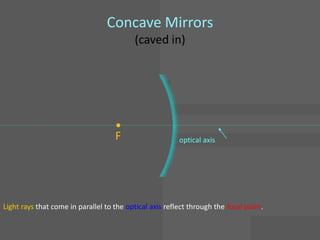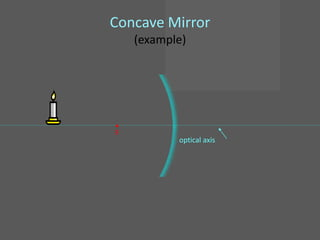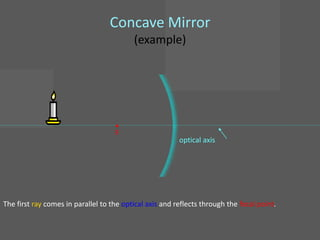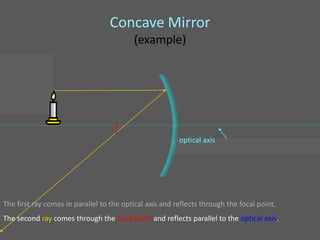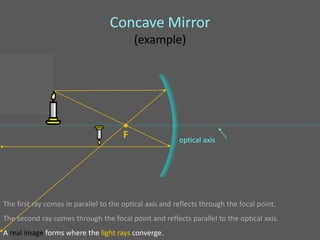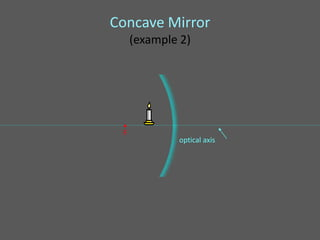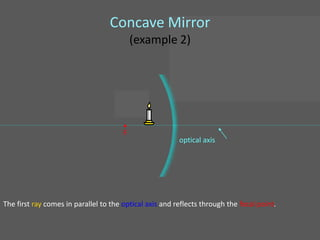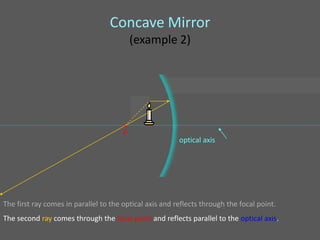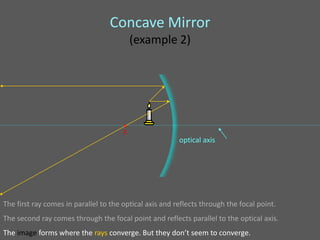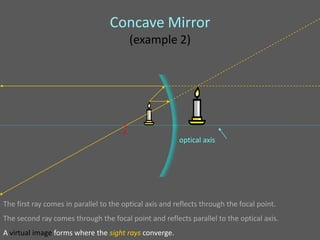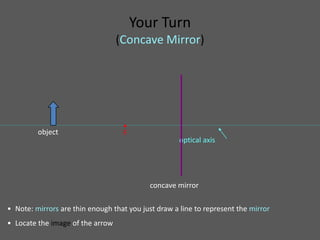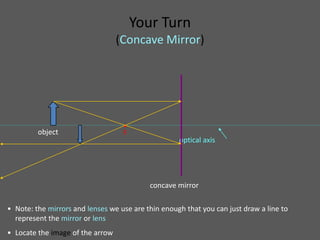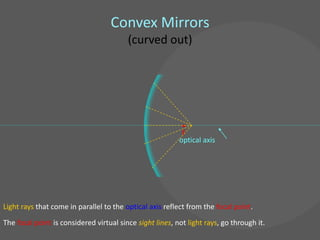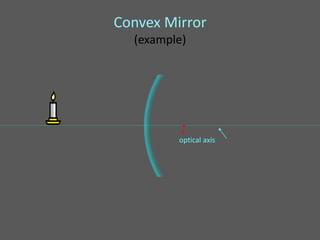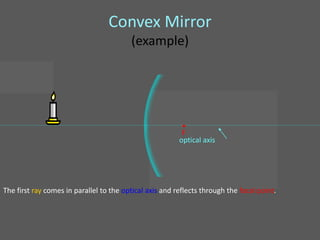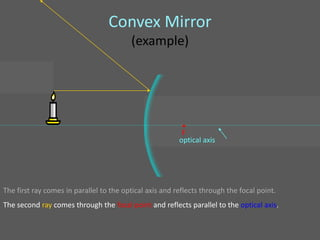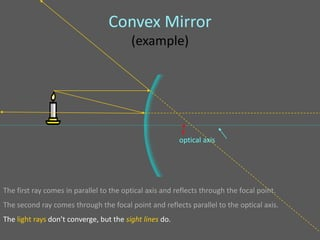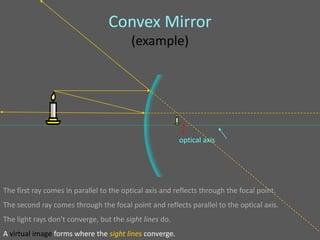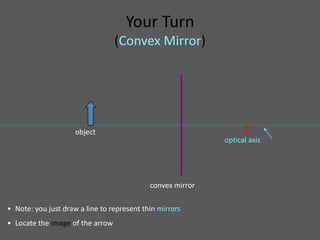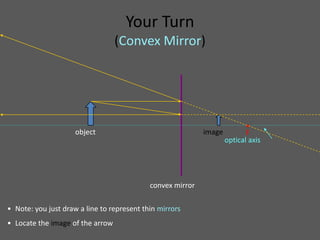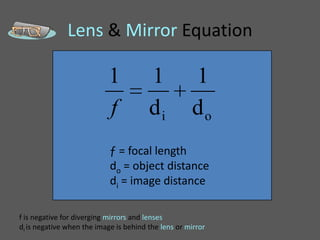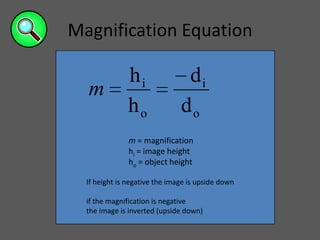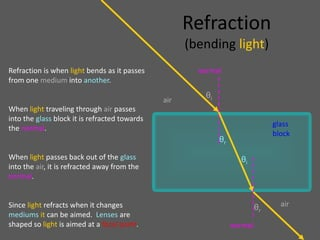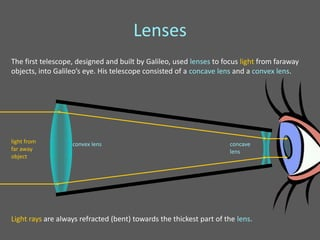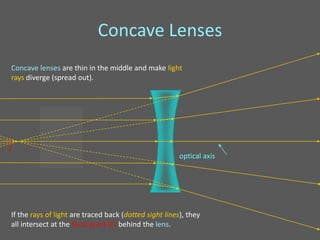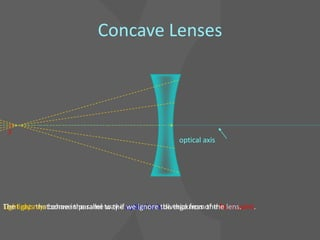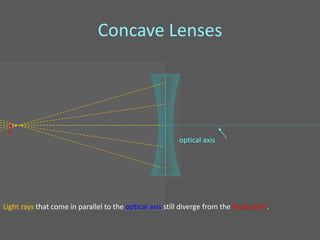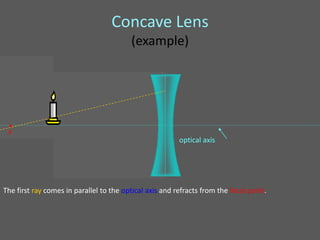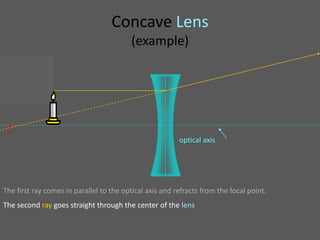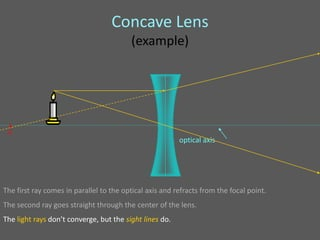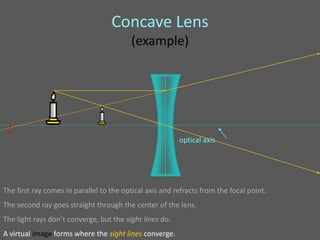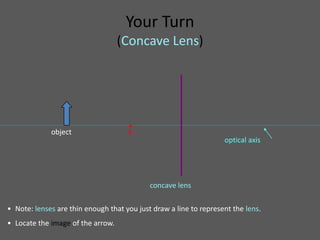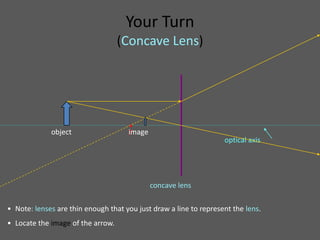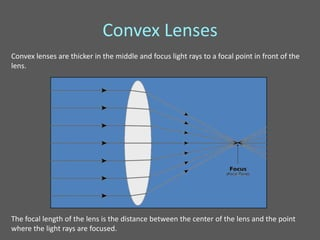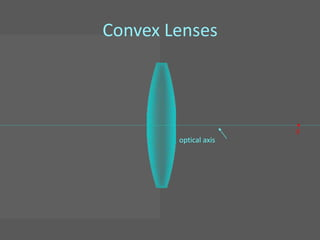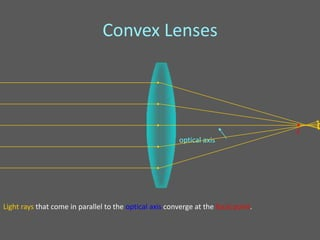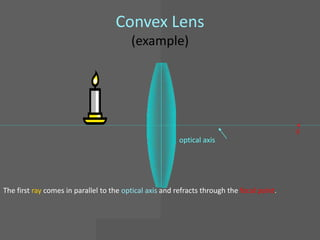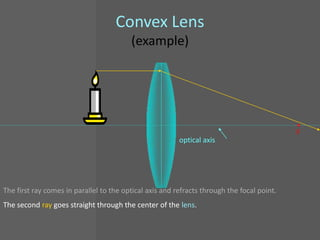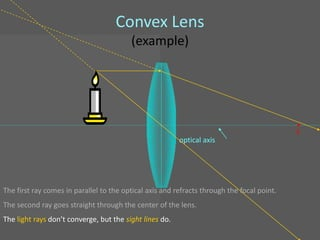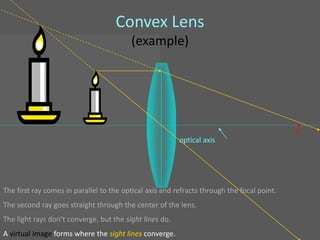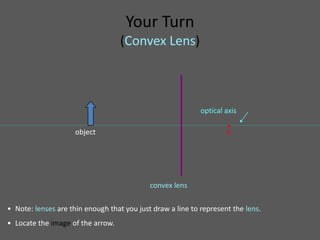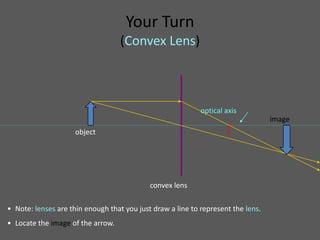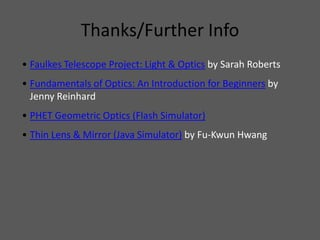1 de 62

### Geometric optics

• 1. Geometric Optics
• 2. Outline • Basics • Reflection • Mirrors • Plane mirrors • Spherical mirrors • Concave mirrors • Convex mirrors • Refraction • Lenses • Concave lenses • Convex lenses
• 3. A ray of light is an extremely narrow beam of light.
• 4. All visible objects emit or reflect light rays in all directions.
• 5. Our eyes detect light rays.
• 6. We think we see objects. We really see images.
• 7. Images are formed when light rays converge. converge: come together
• 8. When light rays go straight into our eyes, we see an image in the same spot as the object. object & image
• 9. Mirrors It is possible to see images when converging light rays reflect off of image mirrors. object
• 10. Reflection (bouncing light) Reflection is when light normal changes direction by bouncing off a surface. When light is reflected off a mirror, it hits the mirror at the same angle (θi, the reflected incident incidence angle) as it reflects ray ray off the mirror (θr, the reflection angle). θr θi The normal is an imaginary line which lies at right angles to the mirror where the ray hits it. Mirror
• 11. Mirrors reflect light rays.
• 12. How do we see images in mirrors?
• 13. How do we see images in mirrors? object image Light from the object reflects off the mirror and converges to form an image.
• 14. Sight Lines object image We perceive all light rays as if they come straight from an object. The imaginary light rays that we think we see are called sight lines.
• 15. Sight Lines object image We perceive all light rays as if they come straight from an object. The imaginary light rays that we think we see are called sight lines.
• 16. Image Types object & object image image window mirror Real images are formed by light rays. Virtual images are formed by sight lines.
• 17. Plane (flat) Mirrors do di ho hi object image Images are virtual (formed by sight lines) and upright Objects are not magnified: object height (ho) equals image height (hi). Object distance (do) equals image distance (di).
• 18. Spherical Mirrors (concave & convex)
• 19. Concave & Convex (just a part of a sphere) r • • C F f C: the center point of the sphere r: radius of curvature (just the radius of the sphere) F: the focal point of the mirror or lens (halfway between C and the sphere) f: the focal distance, f = r/2
• 20. Concave Mirrors (caved in) • F optical axis Light rays that come in parallel to the optical axis reflect through the focal point.
• 21. Concave Mirror (example) • F optical axis
• 22. Concave Mirror (example) • F optical axis The first ray comes in parallel to the optical axis and reflects through the focal point.
• 23. Concave Mirror (example) • F optical axis The first ray comes in parallel to the optical axis and reflects through the focal point. The second ray comes through the focal point and reflects parallel to the optical axis.
• 24. Concave Mirror (example) • F optical axis The first ray comes in parallel to the optical axis and reflects through the focal point. The second ray comes through the focal point and reflects parallel to the optical axis. A real image forms where the light rays converge.
• 25. Concave Mirror (example 2) • F optical axis
• 26. Concave Mirror (example 2) • F optical axis The first ray comes in parallel to the optical axis and reflects through the focal point.
• 27. Concave Mirror (example 2) • F optical axis The first ray comes in parallel to the optical axis and reflects through the focal point. The second ray comes through the focal point and reflects parallel to the optical axis.
• 28. Concave Mirror (example 2) • F optical axis The first ray comes in parallel to the optical axis and reflects through the focal point. The second ray comes through the focal point and reflects parallel to the optical axis. The image forms where the rays converge. But they don’t seem to converge.
• 29. Concave Mirror (example 2) • F optical axis The first ray comes in parallel to the optical axis and reflects through the focal point. The second ray comes through the focal point and reflects parallel to the optical axis. A virtual image forms where the sight rays converge.
• 30. Your Turn (Concave Mirror) • object F optical axis concave mirror • Note: mirrors are thin enough that you just draw a line to represent the mirror • Locate the image of the arrow
• 31. Your Turn (Concave Mirror) • object F optical axis concave mirror • Note: the mirrors and lenses we use are thin enough that you can just draw a line to represent the mirror or lens • Locate the image of the arrow
• 32. Convex Mirrors (curved out) • F optical axis Light rays that come in parallel to the optical axis reflect from the focal point. The focal point is considered virtual since sight lines, not light rays, go through it.
• 33. Convex Mirror (example) • F optical axis
• 34. Convex Mirror (example) • F optical axis The first ray comes in parallel to the optical axis and reflects through the focal point.
• 35. Convex Mirror (example) • F optical axis The first ray comes in parallel to the optical axis and reflects through the focal point. The second ray comes through the focal point and reflects parallel to the optical axis.
• 36. Convex Mirror (example) • F optical axis The first ray comes in parallel to the optical axis and reflects through the focal point. The second ray comes through the focal point and reflects parallel to the optical axis. The light rays don’t converge, but the sight lines do.
• 37. Convex Mirror (example) • F optical axis The first ray comes in parallel to the optical axis and reflects through the focal point. The second ray comes through the focal point and reflects parallel to the optical axis. The light rays don’t converge, but the sight lines do. A virtual image forms where the sight lines converge.
• 38. Your Turn (Convex Mirror) • object F optical axis convex mirror • Note: you just draw a line to represent thin mirrors • Locate the image of the arrow
• 39. Your Turn (Convex Mirror) • object image F optical axis convex mirror • Note: you just draw a line to represent thin mirrors • Locate the image of the arrow
• 40. Lens & Mirror Equation 1 1 1 f di do ƒ = focal length do = object distance di = image distance f is negative for diverging mirrors and lenses di is negative when the image is behind the lens or mirror
• 41. Magnification Equation hi di m ho do m = magnification hi = image height ho = object height If height is negative the image is upside down if the magnification is negative the image is inverted (upside down)
• 42. Refraction (bending light) Refraction is when light bends as it passes normal from one medium into another. θi air When light traveling through air passes into the glass block it is refracted towards glass the normal. block θr When light passes back out of the glass θi into the air, it is refracted away from the normal. Since light refracts when it changes θr air mediums it can be aimed. Lenses are shaped so light is aimed at a focal point. normal
• 43. Lenses The first telescope, designed and built by Galileo, used lenses to focus light from faraway objects, into Galileo’s eye. His telescope consisted of a concave lens and a convex lens. light from convex lens concave far away lens object Light rays are always refracted (bent) towards the thickest part of the lens.
• 44. Concave Lenses Concave lenses are thin in the middle and make light rays diverge (spread out). • F optical axis If the rays of light are traced back (dotted sight lines), they all intersect at the focal point (F) behind the lens.
• 45. Concave Lenses • F optical axis The light that come the same way if we ignore diverge from the focal point. Light raysrays behavein parallel to the optical axisthe thickness of the lens.
• 46. Concave Lenses • F optical axis Light rays that come in parallel to the optical axis still diverge from the focal point.
• 47. Concave Lens (example) • F optical axis The first ray comes in parallel to the optical axis and refracts from the focal point.
• 48. Concave Lens (example) • F optical axis The first ray comes in parallel to the optical axis and refracts from the focal point. The second ray goes straight through the center of the lens.
• 49. Concave Lens (example) • F optical axis The first ray comes in parallel to the optical axis and refracts from the focal point. The second ray goes straight through the center of the lens. The light rays don’t converge, but the sight lines do.
• 50. Concave Lens (example) • F optical axis The first ray comes in parallel to the optical axis and refracts from the focal point. The second ray goes straight through the center of the lens. The light rays don’t converge, but the sight lines do. A virtual image forms where the sight lines converge.
• 51. Your Turn (Concave Lens) • object F optical axis concave lens • Note: lenses are thin enough that you just draw a line to represent the lens. • Locate the image of the arrow.
• 52. Your Turn (Concave Lens) • object image F optical axis concave lens • Note: lenses are thin enough that you just draw a line to represent the lens. • Locate the image of the arrow.
• 53. Convex Lenses Convex lenses are thicker in the middle and focus light rays to a focal point in front of the lens. The focal length of the lens is the distance between the center of the lens and the point where the light rays are focused.
• 54. Convex Lenses • F optical axis
• 55. Convex Lenses • F optical axis Light rays that come in parallel to the optical axis converge at the focal point.
• 56. Convex Lens (example) • F optical axis The first ray comes in parallel to the optical axis and refracts through the focal point.
• 57. Convex Lens (example) • F optical axis The first ray comes in parallel to the optical axis and refracts through the focal point. The second ray goes straight through the center of the lens.
• 58. Convex Lens (example) • F optical axis The first ray comes in parallel to the optical axis and refracts through the focal point. The second ray goes straight through the center of the lens. The light rays don’t converge, but the sight lines do.
• 59. Convex Lens (example) • F optical axis The first ray comes in parallel to the optical axis and refracts through the focal point. The second ray goes straight through the center of the lens. The light rays don’t converge, but the sight lines do. A virtual image forms where the sight lines converge.
• 60. Your Turn (Convex Lens) optical axis • object F convex lens • Note: lenses are thin enough that you just draw a line to represent the lens. • Locate the image of the arrow.
• 61. Your Turn (Convex Lens) optical axis image • object F convex lens • Note: lenses are thin enough that you just draw a line to represent the lens. • Locate the image of the arrow.
• 62. Thanks/Further Info • Faulkes Telescope Project: Light & Optics by Sarah Roberts • Fundamentals of Optics: An Introduction for Beginners by Jenny Reinhard • PHET Geometric Optics (Flash Simulator) • Thin Lens & Mirror (Java Simulator) by Fu-Kwun Hwang